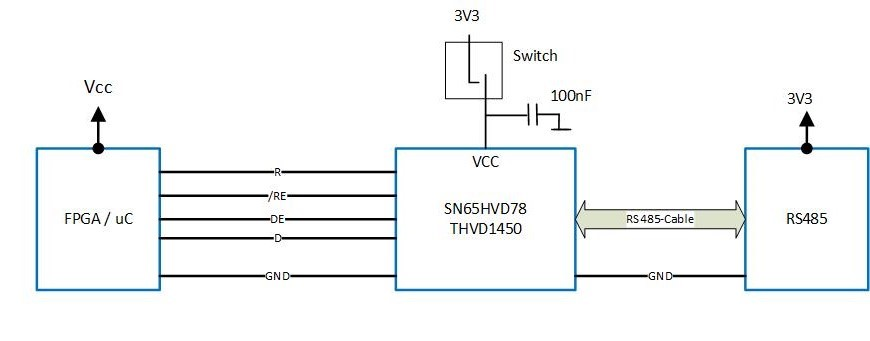If you have a related question, please click the "Ask a related question" button in the top right corner. The newly created question will be automatically linked to this question.

# SN65HVD78: behaviour when supply voltage is switched off

Part Number: SN65HVD78
Other Parts Discussed in Thread: THVD1450

Hello,

my customer would like to use the SN65HVD78 in a new design and had a question:

he would like to know how the device would behave (digital input & output) in case its supply voltage was cut off during normal operation mode (using a load switch for example)?

This is rather not very common but it is something he is foreseeing in his system should there be a fault even for example and he wanted to know how the device would behave.

Thank you.

Regards,

Stani

• Hello,

In some cases abrupt interruptions in current could interact with the parasitic inductance of the power connections to produce spike in the voltage level. If these are large enough, damage to the transceiver may be possible. This would be rare, though, since typically the decoupling capacitance used on the VCC pin would be large enough to suppress the spikes.

Assuming a normal case with no damaging transients, the voltage at VCC would drop (due to the current consumption of the IC). Once the VCC voltage reaches a level at which the transceiver cannot operate (approximately 2.5 V for SN65HVD78), the transceiver would shut off and make its outputs high impedance so that they do not disturb other circuits (for example, the other transceivers that may share the RS-485 bus).

Please let me know if this isn't clear or if you have further questions.

Regards,
Max
• Hi Max,

thank you for your prompt response. I guess the same statement is valid for the THVD1450 right?

Regards,
Stani
• Stani,

Yes, you are correct - the same would apply for THVD1450.

Regards,
Max
• Thanks Max!
• Hi Max,

the customer would like to know what will happen with the input of the transceiver (THVD1450) if the scenario described above occurs?

regards,
Stani
• Stani,

The RS-485 inputs would be high impedance.  To get some idea of the maximum possible leakage currents into the A/B pins in this state, you can refer to the "Bus Input Current" specification in the datasheet.

Regards,
Max

• Hi Max,

thanks.
• Hi Max,

sorry for coming back again. The customer now wants to what the maximum voltage on D, DE, /RE would be when the supply is switched off. Below are the various scenarios he is looking at:

Normal Operation:

VCC = 3,3V / Switch = ON / RE=0V / DE=3,3V / D=3,3V

Scenario 1:

VCC = 3,3V / Switch = OFF / RE=0V / DE=3,3V / D=3,3V

Scenario 2:

VCC = 5,5V / Switch = ON / RE=0V / DE=5,5V / D=5,5V

Scenario 3:

VCC = 5,5V / Switch = OFF / RE=0V / DE=5,5V / D=5,5V

Scenario 4 (defekte Vcc):

VCC = 14V / Switch = OFF / RE=0V / DE=14V / D=14V• Hi Stani,

Is the question concerning the maximum allowed voltage on each pin? If so I would reference the Absolute Maximum Ratings table - these ratings are independent of whether the device is powered on or off.

Or, is the concern with what voltages might couple through the device onto these pins and potentially affect the microcontroller? If that's the case, then the D and RE inputs will track VCC due to the presence of an internal pull-up resistance.

Regards,
Max
• Hi Max,

the concern is your 2nd proposal (what voltage might couple through the device and potentially affect the MCU).

Thanks.

Regards,

Stani